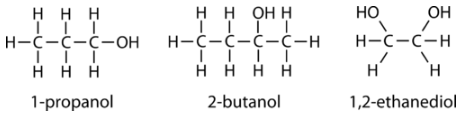7.10: Alcohols

•• Contributed by Elizabeth Gordon
• Lecturer (Chemistry) at Furman University

Water freezes at $$0^\text{o} \text{C}$$ which creates problems for cars in the winter. The water in the engine would freeze and crack the engine block. To prevent this, antifreeze is added to lower the freezing point of the liquid. The most common antifreeze is an alcohol known as propylene glycol. It has largely replaced the much more toxic ethylene glycol. Methanol can also be used as an antifreeze, mainly in windshield wiper fluid.

Alcohols

An alcohol is an organic compound that contains one or more hydroxyl $$\left( \ce{-OH} \right)$$ groups. The general formula for alcohols is $$\ce{R-OH}$$. Do not confuse alcohols with inorganic bases that contain the hydroxide ion $$\left( \ce{OH^-} \right)$$. The $$\ce{-OH}$$ group in an alcohol is covalently bonded to a carbon atom and does not ionize in solution. The steps for naming alcohols are listed below.

1. Name the parent compound by finding the longest continuous carbon atom chain that also contains the hydroxyl group. If there is one hydroxyl group in the molecule, change the final -e in the name of the alkane to -ol. If there is more than one hydroxyl group, use the full name of the alkane and add a suffix to indicate the number of hydroxyl groups. For example, two hydroxyl groups is -diol, three is -triol, etc.
2. Number the carbon chain in a way that makes the sum of the hydroxyl numbers as low as possible.
3. Add the numerical prefix into the name before the name of the alcohol.
4. Separate numbers with commas and separate numbers from names or prefixes with a hyphen. There are no spaces in the name.

Following are three examples of alcohols and their IUPAC names.Aliphatic alcohols can be classified according to the number of $$\ce{R}$$ groups attached to the carbon with the hydroxyl group. If one $$\ce{R}$$ group is attached to that carbon, the alcohol is a primary alcohol. If two $$\ce{R}$$ groups are attached, the alcohol is a secondary alcohol. If three $$\ce{R}$$ groups are attached, the alcohol is a tertiary alcohol. Shown below is an example of each. The primary alcohol is 1-propanol, the secondary alcohol is 2-butanol, and the tertiary alcohol is 2-methyl-2-propanol.Properties of Alcohols

The smallest and lightest alcohols (methanol, ethanol, propanol) are completely soluble in water in all proportions. In a solution, the hydroxyl groups of alcohol molecules and the water molecules form hydrogen bonds with each other, resulting in complete miscibility. However, as the length of the carbon chain increases, the solubility decreases. The solubility of 1-butanol is $$7.4 \: \text{g}$$ per $$100 \: \text{g}$$ of water, while that of 1-pentanol is $$2.7 \: \text{g}$$ per $$100 \: \text{g}$$ of water, and 1-octanol is $$0.06 \: \text{g}$$ per $$100 \: \text{g}$$ of water. The carbon chain portion of the larger alcohol molecule is nonpolar and leads to the decreased solubility of the overall compound.

The presence of hydrogen bonds in alcohols also explains the relatively high boiling points of alcohols compared to the alkanes of similar molar mass (see table below).

Compound Formula Molar Mass $$\left( \text{g/mol} \right)$$ Boiling Point $$\left( ^\text{o} \text{C} \right)$$
Table 25.9.1: Boiling Point Comparison of Alkanes and Alcohols
ethane $$\ce{CH_3CH_3}$$ 32 -88
methanol $$\ce{CH_3OH}$$ 30 64.7
propane $$\ce{CH_3CH_2CH_3}$$ 44 -42.1
ethanol $$\ce{CH_3CH_2OH}$$ 46 78.3

Only weak London dispersion forces hold molecules of nonpolar alkanes together in the liquid phase. Consequently, less energy is required to break these molecules away from the surface of the liquid and turn them into a vapor. The stronger hydrogen bonding between alcohol molecules means that more energy is required to convert the liquid to vapor, and boiling points are therefore high.

This page briefly discusses some of the important uses of simple alcohols, such as methanol, ethanol and propan-2-ol.

Alcoholic Drinks

The word "alcohol" in alcoholic drinks refers to ethanol (CH3CH2OH).

Industrial methylated spirits

Ethanol is usually sold as industrial methylated spirits, which is ethanol with a small quantity of methanol and possibly some color added. Because methanol is poisonous, industrial methylated spirits are unfit to drink, allowing purchasers to avoid the high taxes levied on alcoholic drinks.

Use of ethanol as a fuel

Ethanol burns to produce carbon dioxide and water, as shown in the equation below, and can be used as a fuel in its own right or in mixtures with petrol (gasoline). "Gasohol" is a petrol/ethanol mixture containing approximately 10–20% ethanol. Because ethanol can be produced by fermentation, this is a useful method for countries without an oil industry to reduce the amount of petrol imports.

$CH_3CH_2OH +3O_2 \rightarrow 2CO_2 + 3H_2O$

Ethanol as a solvent

Ethanol is widely used as a solvent. It is relatively safe and can be used to dissolve many organic compounds that are insoluble in water. It is used, for example, in many perfumes and cosmetics.

Methanol as a fuel

Methanol also burns to form carbon dioxide and water:

$2CH_3OH +3O_2 \rightarrow 2CO_2 + 4H_2O$

It can be used a a petrol additive to improve combustion, and its use as a fuel in its own right is under investigation.

Methanol as an industrial feedstock

Most methanol is used to make other compounds, for example, methanal (formaldehyde), ethanoic acid, and methyl esters of various acids. In most cases, these are then converted into further products.